### Introduction

Abstraction: Describing a system; input, output
Mathematics:
1.Solving differiential equations.

Ex1:

2.Geometric sum

Ex2: Use Taylor or division method.

3.Partial fraction
4.Multiplying polynomials (e.g. tabular representation)
5.Complex Numbers: $e^{j\theta} = \cos\theta+j\sin\theta$

CT transformation: odd/even, shift, scaling…

### DT Systems

Unit sample:

Operator: $\mathcal{R}$, right-shift operator
Fundamental modes: e.g. $y[n]=(0.5)^n$

Multiple representations:

• Verbal description
• Difference equation: precise and concise
• Block diagram: (start at rest) trace the flow of information
• step-by-step
• operator approach, polynomials
Lumping: from samples to signals
Declarative vs. Imperative (equation; diagram)
Recipe vs. Constraint
Acyclic vs. Cyclic
Finite vs. Infinite response

#### Standard form (DT)

Geometric growth: pole (unit-sample response)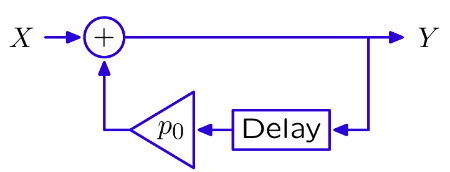converge: |p|<1;   diverge: |p|>1

Second-Order System: poles (still unit-sample)
see the Ex3.
two methods: multipying polynomial; partial fraction

Complex poles:
Thinking about the difference equations, if the coefficients are real, even though the roots may be complex, the output is still real.
Note: visualize the complex plane.

Summary:
Systems composed of adders, gains, and delays can be characterized by their poles.
The poles of a system determine its fundamental modes.
The unit-sample response of a system can be expressed as a weighted sum of fundamental modes.

#### Z tranform

Replace $\mathcal{R}$ with $z^{-1}$.
Def: (bilateral)

Region of Convergence (ROC)
Note: For z transform, always consider both the functional form and the region of convergence.
Properties:
1.Linearity
2.Delay property   $x[n-1]\leftrightarrow z^{-1}X(z)$ for $z$ in ROC.
IMPORTANT :
right-sided signal $\rightarrow$ outside region
IF inside region, solve the difference equation by iterating backwards in time.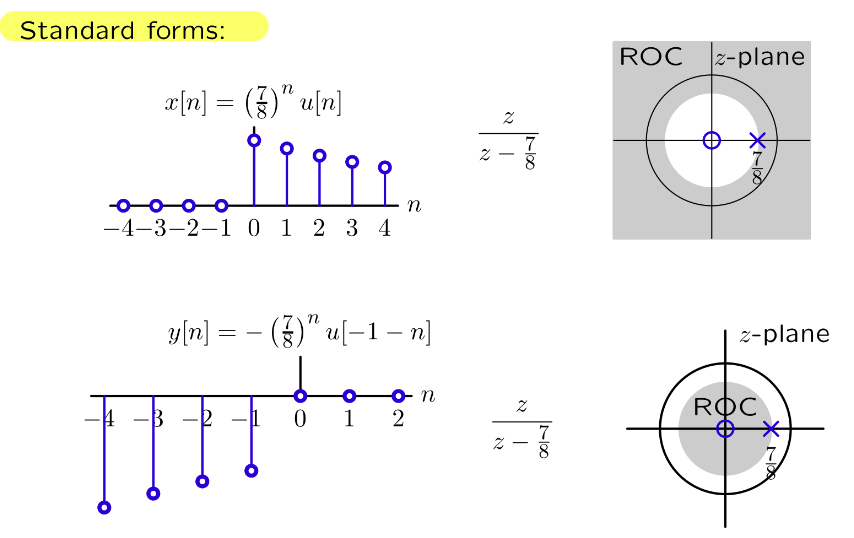Inverse transform:
Formally, $x[n]=\frac{1}{2\pi j}\int_CX(z)^{n-1}dz$
Better ways: partial fractions; using $\mathcal{R}$,….

### CT Systems

Unit impulse:
Unit area but zero width. (represented by an arrow with the number 1)
Unit step:
$u(t)=\int_{-\infty}^{t}\delta(t)dt = 1 \ (\text{only when }t\geq 0)$
Operator: $\mathcal{A}$, accumulator, $Y=\mathcal{A}X$ means $y(t)=\int_{-\infty}^{t}x(t)dt$

Fundamental operation:  Delays in DT are replaced by integrators in CT.

#### Standard form (CT)

Methods for solving CT Systems:
1.differential equation $\rightarrow$ solve it;
2.use operators (Using poles; or Taylor series)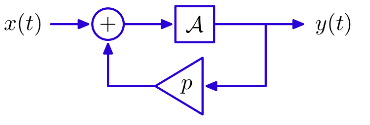converge: p<0; diverge: p>0;

#### Laplace tranform (CT)

Def: (bilateral)

ROC: Thinking about convergence, only thinking about the real part.
$e^{pt}=e^{(\sigma+jw)t}= e^{\sigma t}(\cos wt+j\sin wt)$
right-sided signal $\rightarrow$ right-sided region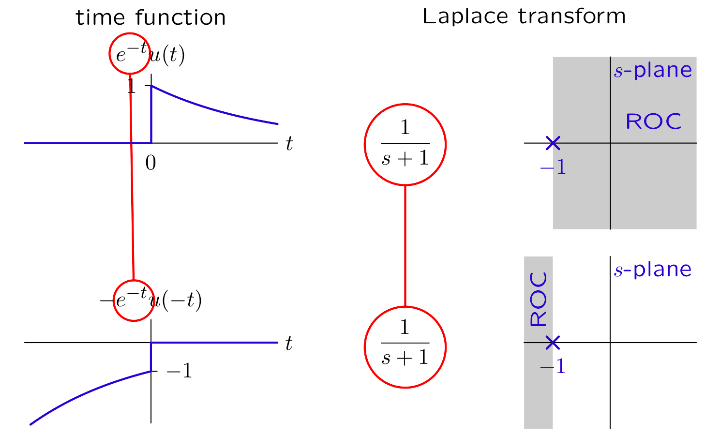Solving Differential equations with Laplace transform:
Laplace transform of the derivative $\rightarrow$ s times the L-transform of the original function.

### Summary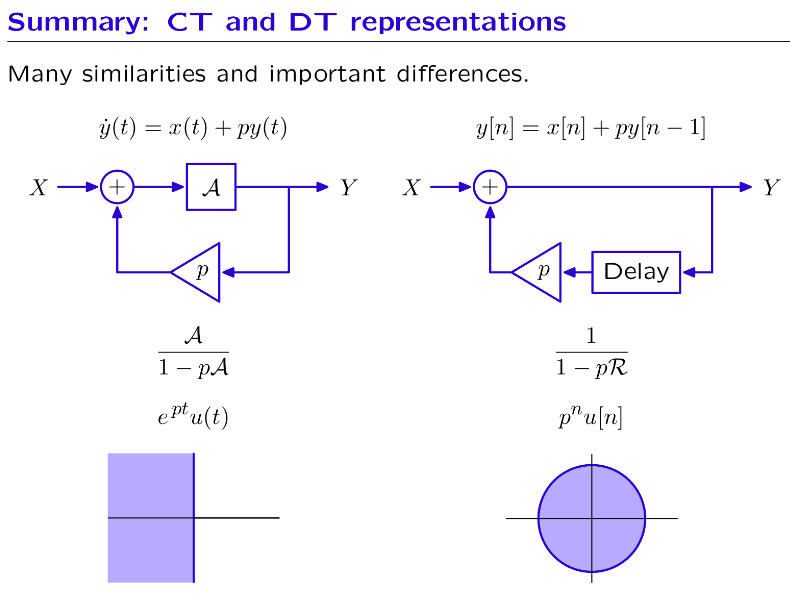Connections: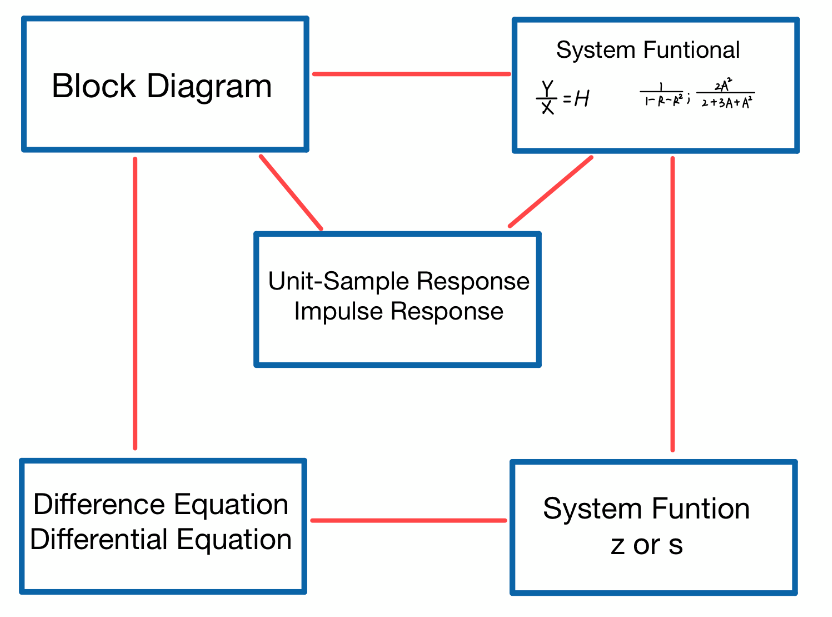### Examples

Ex3: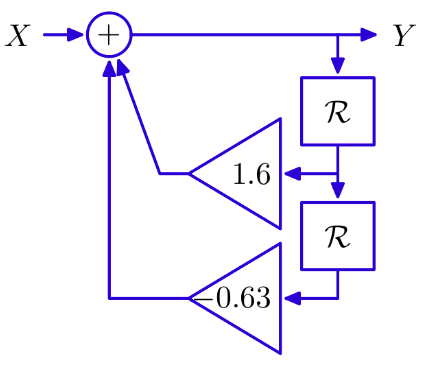Note: 可以将原来的diagram化成两个simpler system,cascaded system

Method 1: Multiplying polynomials \begin{aligned} \frac{Y}{X} &=(1+0.7\mathcal{R}+0.7^2\mathcal{R^2}+\cdots)(1+0.9\mathcal{R}+0.9^2\mathcal{R^2}+\cdots)\\ &=1+(0.7+0.9)\mathcal{R}+(0.7^2+0.7\times0.9+0.9^2)\mathcal{R^2}+\dots\end{aligned}   Note: 可以用tabular
Method 2:
Partial Fraction \begin{aligned} \frac{Y}{X}&=\frac{4.5}{1-0.9\mathcal{R}}-\frac{3.5}{1-0.7\mathcal{R}}\\ \text{if } x[n] &= \delta[n],\ \text{then }\ y[n] = 4.5(0.9)^n-3.5(0.7)^n \text{ for n\geq0.} \end{aligned} Check:

Ex4: Find the poles of Fibonacci system.
Key: difference equation: $y[n] = y[n-1]+y[n-2]+x[n]$
poles: 1.618… and -0.618…

PS：可能不会再有了吧！
这几百行打下来也太太太太费劲了吧！
敲公式真的活生生敲出概率论作业的感jio啊！！！
也只有在疫情爆发不能出门的日子里
脑子抽了才会想整这个吧orz